# TableCurve 2D - Product UsesAn example is the analysis of regional pulmonary ventilation of a 133Xe airway bolus using a scintillation camera. Since the bolus of xenon initially washes into the lung and subsequently washes out, we would expect one of the TableCurve intermediate kinetic functions to fit the data. See the TableCurve PDF nonlinear equation documentation for description of the intermediate functions. They represent measurement from the middle of three compartments. In our case the middle compartment is the lung with the airway and recirculation-via-organ-systems being the first and third compartments, respectively. Six of ten measured regions are shown in Figure 1. We want to fit all ten data sets as a batch and place the results in a Word file.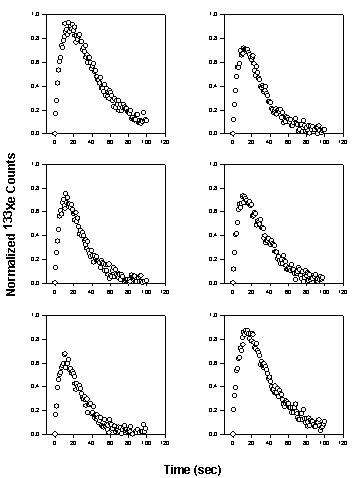### Setting Up Automation

This is a two step procedure:

1. select options to fit the first data set
2. use these options for Automation to fit all data sets

### Fit the First Data Set

You fit the first data set exactly the same way you normally use TableCurve except in this case we are going to restrict the fitting to the Kinetic equations. Open TableCurve and Import the file regional 133Xe washout.xls that you have downloaded. The Select Columns dialog, Figure 2, shows the data in columns A K of the Excel worksheet. This data is in X Many Y format with the X data in column A and the ten Y data sets in columns B – K.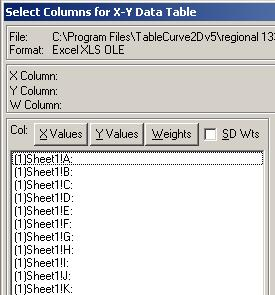Figure 2. Select Columns dialog with time data in column A and ten regional scintillation measurements in columns B through K.

Select column A and B to be the X and Y data. At the Process menu select Edit Custom Equation Set. For each tab select Clear to deselect the equations except for the Kinetic tab – select all Kinetic equations. The dialog for the Kinetic tab is shown in Figure 3.Figure 3. Kinetic tab of the Custom Equations dialog. All functions are selected. All functions in all other tabs are deselected.

Click the Fit button shown in Figure 3 to fit these equations to the data in columns A and B of the Excel worksheet. Click Graph Start to view the Review Curve Fit window and equations. The equation list shows the highest ranked equation (by either R2 or F-Statistic) is “Intermed11 c < d(a,b,c,d)” which is what we expected. For this data set the Equil111 equations are probably not significantly different from the Intermed11 equations.Figure 4. Equation list ranked by the F-statistic. The Intermed11 equations fit best.

### Use Automation to Fit All Data Sets

We will now use Automation to fit all ten data sets with the equation selected to be the best during the fit of the first data set (Intermed11 c < d(a,b,c,d)) and place the results in a Word document. The Automation icon is located at the bottom of the icon collection of the Review Curve-Fit window. Click on it to obtain the Automation dialog.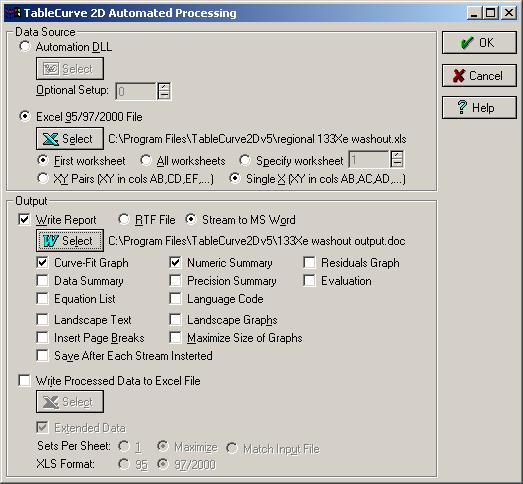Figure 5. The Automation dialog. The data source is the Excel file ‘regional 133Xe washout.xls’. The Word output file is ‘133Xe washout output.doc’. Two output options, Curve-Fit Graph and Numeric Summary, are selected to view the curve fit results and obtain numeric parameter values for the ten data sets.

Our data source is an Excel file and our data is located in the first worksheet Single X (XY in cols AB, AC, AD,…) format. These are the options selected in the Data Source group box in Figure 5.

Select the options in the Output group box in Figure 5 to put the ten data set curve fit results in the Word document ‘133Xe washout output.doc’.

Click OK to curve fit the ten data sets with the Intermed11 c < d(a,b,c,d) equation.

### Results of the Automated Fit

Graphs for the fit to each data set and the numerical fit results are placed into the Word document. The results for the second data set are representative and are shown in Figure 6.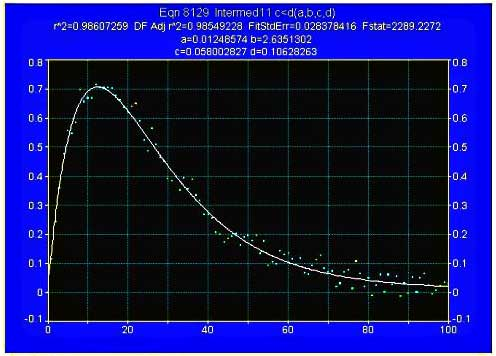Figure 6. Automated results in the Word document for the second data set.

Rank 1 Eqn. 8129 Intermed11 c < d ( a,b,c,d)

 r2 Coef Det DF Adj r2 Fit Std Err F-value 0.9860725867 0.9854922778 0.0283784161 2289.2272163

Parm Value Std Error t-value 95% Confidence Limits P>|t|
a. 0.012485740 0.009232193 1.352413322 -0.00583761 0.030809087 0.17939
b. 2.635130203 0.450727827 5.846389021 1.740560240 3.529700167 0.00000
c. 0.058002827 0.007593421 7.638563579 0.042931989 0.073073665 0.00000
d. 0.106282625 0.014492888 7.333433025 0.077518254 0.135046997 0.00000

An excellent fit is obtained with and F ratio > 2000. Of physiological interest, the clearance constant (c parameter) is 0.058 sec-1. For all data sets this clearance rate ranges from 0.028 to 0.065 sec-1 with corresponding time constants of 35 (apical lung region) to 15 seconds (basal lung region).

### Processing TableCurve Results

The Word file is an excellent report format but if you want to continue to process the results of TableCurve Automation then use the Excel output option.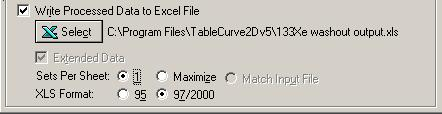Figure 7. Options to place TableCurve Automation results into an Excel file.

The options shown in Figure 7 will place the fit results of the ten data sets into ten worksheets in the Excel file "133Xe washout output.xls". You can then write a simple VBA macro in Excel to process these results. For example, you might create a table of lung clearance constant (c parameter) results for the ten data sets as a function of distance from lung apex to base.TableCurve 2D and SigmaScan Pro were used to characterize the size distribution of oil droplets. SigmaScan measured the oil droplet radius and TableCurve® found the Weibull function to best characterize their size distribution. Oil droplets, suspended in a fluid column were imaged using conventional CCD camera technology and a PC compatible frame grabber board. The image shown in Figure 1 is: an image of oil droplets suspended in a fluid column.Figure 2: Image after contrast enhancement. The image was calibrated using a two point calibration from the Calibrate, Distance and Area option in the Image menu. The contrast was enhanced using the Histogram Stretch procedure (Image, Intensity menu) so that operators could better visualize the oil droplets (the Old Start line end point was dragged with the mouse to intensity 192 which stretches the light gray (192) to white (255) range over then entire 0 – 255 range). The enhanced image is shown in Figure 2.Figure 3: Selecting the oil droplets using intensity thresholding. Intensity Thresholding the image in the intensity range 0 – 140 using the Image, Threshold option selected the darker oil droplets. The selected oil droplets are shown in the red overlay plane in Figure 3.Figure 4: Using the Fill Holes procedure to eliminate open areas in the oil droplets. Due to the surface reflections, intensity thresholding will not select all pixels in some of the oil drops. The Fill Holes feature in the Image, Overlay Filters dialog was used to allow accurate droplet area measurements to be made. This fills the holes in the droplets as shown in Figure 4.

### Measurements and Results

The objects on the red overlay plane were then counted and the parameters perimeter, area, ferret diameter, shape factor, compactness and number of pixels measured using the Measure Objects option in the Measurements menu. These measurements were selected from the list in the Measurements tab in the Measurements Settings dialog. A macro was written to compute the circular radius of each droplet using the equation R = (A/pi)^0.5 and the results placed into the worksheet. A histogram of droplet radius from 0 to 10 microns was also computed. An ASCII file report was generated and formatted in Excel.

 Perimeter Area Feret Diameter Shape Factor Compact # Pixels Radius Size Range Number of Droplets 9.57 6.99 2.98 0.96 13.11 16 1.49 0 0 1.87 0.44 0.75 1.57 8 1 0.37 1 51 9.96 6.11 2.79 0.77 16.22 14 1.39 2 37 66.91 63.31 14.44 0.46 27.41 374 7.21 3 30 15.57 17.03 4.66 0.88 14.23 39 2.33 4 13 31.91 34.5 6.63 0.43 29.51 79 3.31 5 6 25.53 19.65 5 0.38 33.16 45 2.5 6 2 3.19 0.87 1.05 1.08 11.66 2 0.53 7 3 1.87 0.44 0.75 1.57 8 1 0.37 8 1 14.63 15.28 4.41 0.9 14.01 35 2.21 9 0 1.87 0.44 0.75 1.57 8 1 0.37 10 0 14.08 10.04 3.58 0.64 19.75 23 1.79 14.86 11.35 3.8 0.65 19.45 26 1.9 19.53 19.21 4.95 0.63 19.85 44 2.47 14.08 10.92 3.73 0.69 18.17 25 1.86

The histogram data in the last two columns was copied into TableCurve 2D. All peak functions were selected from the Custom Equation dialog and the Weibull distribution found to fit the data best. The TableCurve® graph of these results is shown in Figure 5 below:PACT95, the coalition that designed the fastest American yacht in the 1995 America’s Cup race, Young America, chose TableCurve 2D to help optimize their design because it is the only software that, in seconds, searches through thousands of equations to describe difficult-to-model empirical data. Taking data samples from numerous sources, including wind tunnel tests, tank testing, and data from many other types of experiments, TableCurve® was able to convert vast amounts of empirical data into simplified curve fits. These algorithms were then input into a Velocity Prediction Program (VPP) that balances the equations of motion while looking for the fastest boat.

A closed form expression for F cannot be obtained for the Baker and Lonsdale equation. However, since the expression for F does not contain the parameter k, it can be numerically ‘inverted’. The numerical inversion was done with TableCurve 2D®.

A closed form expression for F cannot be obtained for the Baker and Lonsdale equation (first equation in the Table). However, since the expression for F does not contain the parameter k, it can be numerically ‘inverted’. The numerical inversion was done with TableCurve 2D® using the following procedure. First the expression for F on the left side of the equation was evaluated for 1000 equidistant F values from 0 to 100. The columns containing the expression for F (which equals kt) and F were then reversed so that F is now the Y variable and kt is the X variable. All equations in TableCurve were then fit to this X,Y data set and the equations ranked using the F statistic. The best fitting equation found (Fstat = 2.7×1014) was the following rational polynomial in fractional powers of x.where the coefficients are

a = 2.5788672e-6

b = -3.4434044

c = 244.94883

d = 3.9105658

e = -976.78997

f1= -1.5002823

g = 1407.9333

h = 0.039306878

i = -862.63205

j = 0.0091845726

k1=187.88278

In this equation F is an explicit function of x (=kt) so it is used in the nonlinear curve fitter to estimate the parameter k. An analysis of the residuals found the maximum absolute difference between the actual F and approximate F to be 0.0003 (at F= 99.9). The maximum absolute percentage difference was only 0.002% (at F = 0.1).